# Selva Prabhakaran

Selva is the Chief Author and Editor of Machine Learning Plus, with 4 Million+ readership. He has authored courses and books with100K+ students, and is the Principal Data Scientist of a global firm.## Caret Package – A Practical Guide to Machine Learning in R

Caret Package is a comprehensive framework for building machine learning models in R. In this tutorial, I explain nearly all the core features of the caret package and walk you through the step-by-step process of building predictive models. Be it a decision tree or xgboost, caret helps to find the optimal model in the shortest …## Numpy Tutorial Part 2 – Vital Functions for Data Analysis

Numpy is the core package for data analysis and scientific computing in python. This is part 2 of a mega numpy tutorial. In this part, I go into the details of the advanced features of numpy that are essential for data analysis and manipulations. Introduction How to get index locations that satisfy a given condition …## Numpy Tutorial – Your first numpy guide to build python coding foundations

This is part 1 of the numpy tutorial covering all the core aspects of performing data manipulation and analysis with numpy’s ndarrays. Numpy is the most basic and a powerful package for scientific computing and data manipulation in python. Numpy Tutorial Part 1: Introduction to Arrays. Photo by Bryce Canyon. Also Read: Numpy Tutorial – …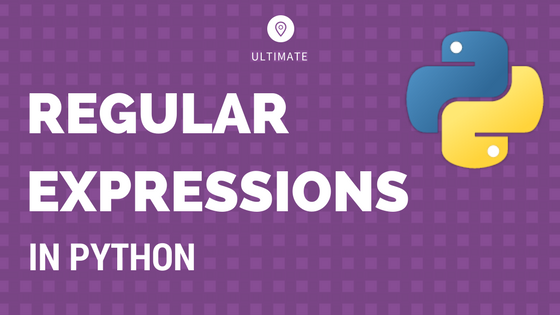## Python Regular Expressions Tutorial and Examples: A Simplified Guide

Regular expressions, also called regex, is a syntax or rather a language to search, extract and manipulate specific string patterns from a larger text. It is widely used in projects that involve text validation, NLP and text mining Regular Expressions in Python: A Simplified Tutorial. Photo by Sarah Crutchfield. Contents 1. Introduction to regular expressions …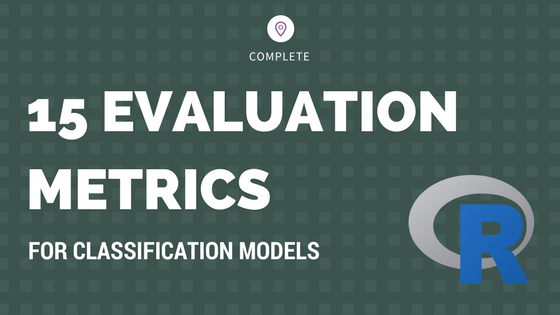## Evaluation Metrics for Classification Models – How to measure performance of machine learning models?

Choosing the right evaluation metric for classification models is important to the success of a machine learning app. Monitoring only the ‘accuracy score’ gives an incomplete picture of your model’s performance and can impact the effectiveness. So, consider the following 15 evaluation metrics before you finalize on the KPIs of your classifier model. Introduction: Building …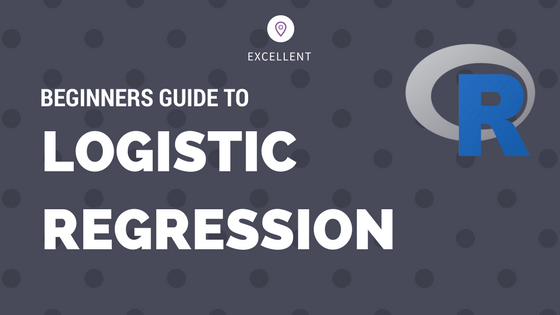## Logistic Regression – A Complete Tutorial With Examples in R

Logistic regression is a predictive modelling algorithm that is used when the Y variable is binary categorical. That is, it can take only two values like 1 or 0. The goal is to determine a mathematical equation that can be used to predict the probability of event 1. Once the equation is established, it can …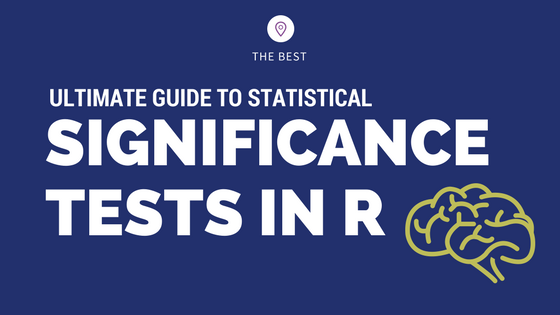## How to implement common statistical significance tests and find the p value?

How to implement and interpret the commonly used statistical significance tests in R? Understand the purpose, when to use and how to interpret the test results and the p value. Correlation Test and Introduction to p value One Sample t-Test Wilcoxon Signed Rank Test Two Sample t-Test and Wilcoxon Rank Sum Test Shapiro Test Kolmogorov …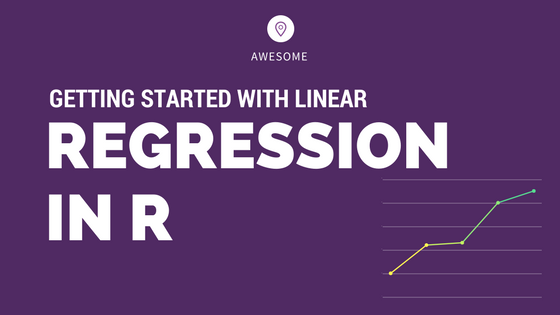## Complete Introduction to Linear Regression in R

We have covered the basic concepts about linear regression. Besides these, you need to understand that linear regression is based on certain underlying assumptions that must be taken care especially when working with multiple Xs. Once you are familiar with that, the advanced regression models will show you around the various special cases where a …Course Preview

## Machine Learning A-Z™: Hands-On Python & R In Data Science

### Free Sample Videos:#### Machine Learning A-Z™: Hands-On Python & R In Data Science#### Machine Learning A-Z™: Hands-On Python & R In Data Science#### Machine Learning A-Z™: Hands-On Python & R In Data Science#### Machine Learning A-Z™: Hands-On Python & R In Data Science